# Basic functions - math word problems

#### Number of problems found: 2267

• School marks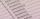Boris has a total of 22 marks. Ones have 3 times less than threes. There are two twos. How many has ones and threes?
• Efficiency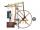A certain device consumes 28 kW and delivers 14 kW. Find its efficiency.
• Trapezoid - central medianThe central median divides the trapezoid into two smaller trapezoids. Determines the ratio of their contents.
• If you 3If you deposit \$4500 at 5% annual interest compound quarterly, how much money will be in the account after 10 years?
• DecibelBy what percentage does the sound intensity increase if the sound intensity level increases by 1 dB?
• Altitude difference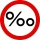What a climb in per mille of the hill long 4 km and the altitude difference is 6 meters?
• Efficiency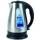What is the power output of kettle 2 kW with efficiency 90%?
• DivisibilityWrite all the integers x divisible by seven and eight at the same time for which the following applies: 100
• LiftThe largest angle at which the lift rises is 16°31'. Give climb angle in permille.
• MATES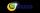In MATES (Small Television tipping) from 35 randomly numbers drawn 5 winning numbers. How many possible combinations there is?
• SequenceIn the arithmetic sequence is a1=-1, d=4. Which member is equal to the number 203?
• The angles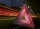The angles in the triangle are in the ratio 12: 15: 9. Find the angles.
• Highway repairThe highway repair was planned for 15 days. However, it was reduced by 30%. How many days did the repair of the highway last?
• How manyHow many numbers are less than 222 with a digit sum is 8?
• Two ages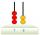Gabrielle is 9 years younger than Mikhail. The sum of their ages is 87. What is Mikhail's age?
• If weIf we increase the unknown number by 4%, we get 780. Determine the unknown number.
• Points in planeThe plane is given 12 points, 5 of which is located on a straight line. How many different lines could by draw from this points?
• EnrollmentThe enrollment at a local college increased 4% over last year's enrollment of 8548. Find the increase in enrollment (x1) and the current enrollment (x2).
• MidnightHow many hours are, if the time that elapsed since 8:00 is 2/5 of the time that will past till midnight?
• Company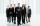After increasing the number of employees by 15% company has 253 employees. How many employees take?

Do you have an interesting mathematical word problem that you can't solve it? Submit a math problem, and we can try to solve it.

We will send a solution to your e-mail address. Solved examples are also published here. Please enter the e-mail correctly and check whether you don't have a full mailbox.

Please do not submit problems from current active competitions such as Mathematical Olympiad, correspondence seminars etc...